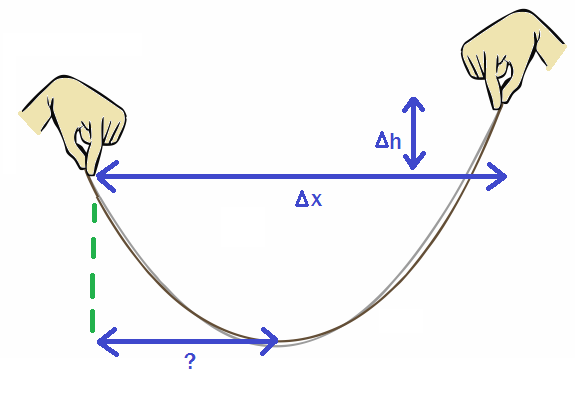# Catenary?

Geometry Level 2If you dangle a chain, as shown in the picture above, it forms a hyperbolic cosine function.

Assume the following values:

• $\Delta h = 6$ inches
• $\Delta x = 2$ feet.

What is the distance from the left (lower) side to the lowest point of the chain, in inches?

Give your answer to $2$ decimal places.

If you think the answer can't be determined, please put 99999.

Image credit: https://methmath.wordpress.com

×

Problem Loading...

Note Loading...

Set Loading...